# Finite Math Pro

• 0 votes
Price \$0.99 Education 1.4 Larry Feldman Jul 19th, 2013 0 votes 1

You’re taking Finite Mathematics, and you don’t have this app? Give yourself an edge! "Finite Math Pro" contains a rich collection of tutorials, examples, and solvers for the following topics: Points and Lines: – Solvers and tutorials to find slopes, intercepts, equations of lines, and graphs of lines – System solver for 2 variables and 2 equations – Tutorials for solving systems by graphing, substitution, and linear combinations Linear Regression: – Solver to find the best fit line and correlation coefficient for up to 30 points – Linear regression tutorial and example Matrices: – Adding, subtracting, and multiplying matrices – Identity matrices- Solvers and tutorials to find determinants/inverses of 2 x 2 and 3 x 3 matrices Linear Programming (graphical method): – Tutorial – Example with step-by-step solution Probability: – Formulas – Survey Results – Venn Diagrams- Bayes’ Theorem- Permutations – Combinations – Balls in Urns- Independent Events- Poker, Roulette, Dice, Lottery, General Card ProblemsNormal Distributions: – Solve for area under a bell curve without using Z-score tables – Solve for value on bell curve given area without using Z-score tables – Examples and tutorials Compound Interest: – Solve for amount, principle, time or rate – Examples and tutorials If you like my app, please leave a review! If you don’t like my app, please email me with your constructive feedback!I welcome all comments, questions, and suggestions:[email protected] will answer your math questions for free on facebook:http://www.facebook.com/lfeldmantutoringPlease check out my other Android apps:Algebra ProACT Math PrepCalculus ProGeometry ProMath Pro (6 math apps for \$1.99!)PreCalculus ProProbability ProSAT Math PrepStatistics Pro

## App Screenshots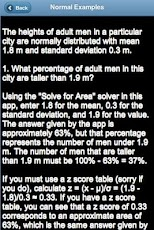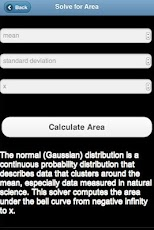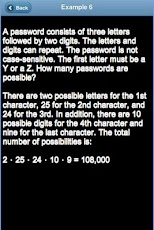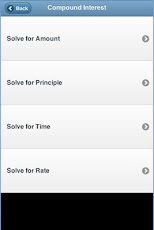## Other Education Apps#### iLiveMath3 – 3r...

(0)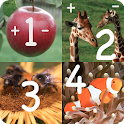#### iLiveMath1234 Lite

(0)#### iLiveMath1 – 1s...

(0)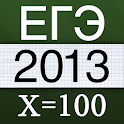#### ЕГЭ 2013. Мате...

(66)#### Guide Peru

(0)(0)

## Comments

Start typing and press Enter to search